Search

Conics: Hyperbolas: Introduction (page 1 of 3)

Sections: Introduction, Finding information from the equation, Finding the equation from informationHyperbolas don't come up much  at least not that I've noticed  in other math classes, but if you're covering conics, you'll need to know their basics. An hyperbola looks sort of like two mirrored parabolas, with the two "halves" being called "branches". Like an ellipse, an hyperbola has two foci and two vertices; unlike an ellipse, the foci in an hyperbola are further from the hyperbola's center than are its vertices: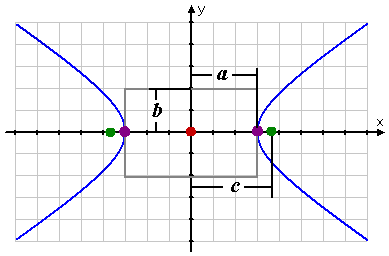The hyperbola is centered on a point (h, k), which is the "center" of the hyperbola. The point on each branch closest to the center is that branch's "vertex". The vertices are some fixed distance a from the center. The line going from one vertex, through the center, and ending at the other vertex is called the "transverse" axis. The "foci" of an hyperbola are "inside" each branch, and each focus is located some fixed distance c from the center. (This means that a < c for hyperbolas.) The values of a and c will vary from one hyperbola to another, but they will be fixed values for any given hyperbola.

For any point on an ellipse, the sum of the distances from that point to each of the foci is some fixed value; for any point on an hyperbola, it's the difference of the distances from the two foci that is fixed. Looking at the graph above and letting "the point" be one of the vertices, this fixed distance must be (the distance to the further focus) less (the distance to the nearer focus), or (a + c)  (c  a) = 2a. This fixed-difference property can used for determining locations: If two beacons are placed in known and fixed positions, the difference in the times at which their signals are received by, say, a ship at sea can tell the crew where they are.

As with ellipses, there is a relationship between a, b, and c, and, as with ellipses, the computations are long and painful. So trust me that, for hyperbolas (where a < c), the relationship is c2  a2 = b2 or, which means the same thing, c2 = b2 + a2. (Yes, the Pythagorean Theorem is used to prove this relationship. Yes, these are the same letters as are used in the Pythagorean Theorem. No, this is not the same thing as the Pythagorean Theorem. Yes, this is very confusing. Just memorize it, and move on.)

 When the transverse axis is horizontal (in other words, when the center, foci, and vertices line up side by side, parallel to the x-axis), then the a2 goes with the x part of the hyperbola's equation, and the y part is subtracted.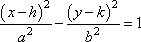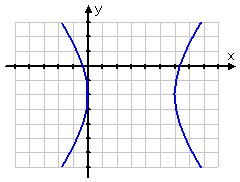When the transverse axis is vertical (in other words, when the center, foci, and vertices line up above and below each other, parallel to the y-axis), then the a2 goes with the y part of the hyperbola's equation, and the x part is subtracted.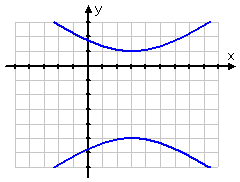In "conics" form, an hyperbola's equation is always "=1".

The value of b gives the "height" of the "fundamental box" for the hyperbola (marked in grey in the first picture above), and 2b is the length of the "conjugate" axis. This information doesn't help you graph hyperbolas, though. Copyright © Elizabeth Stapel 2010-2011 All Rights Reserved

For reasons you'll learn in calculus, the graph of an hyperbola gets fairly flat and straight when it gets far away from its center. If you "zoom out" from the graph, it will look very much like an "X", with maybe a little curviness near the middle. These "nearly straight" parts get very close to what are called the "asymptotes" of the hyperbola. For an hyperbola centered at (h, k) and having fixed values a and b, the asymptotes are given by the following equations:

 hyperbolas' graphs asymptotes' equations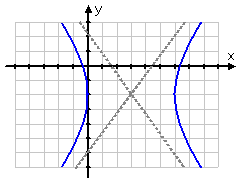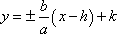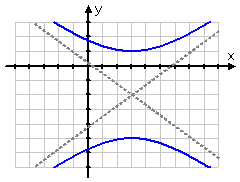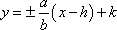Note that the only difference in the asymptote equations above is in the slopes of the straight lines: If a2 is the denominator for the x part of the hyperbola's equation, then a is still in the denominator in the slope of the asymptotes' equations; if a2 goes with the y part of the hyperbola's equation, then a goes in the numerator of the slope in the asymptotes' equations.

Hyperbolas can be fairly "straight" or else pretty "bendy":

 hyperbola with aneccentricity of about 1.05 hyperbola with aneccentricity of about 7.6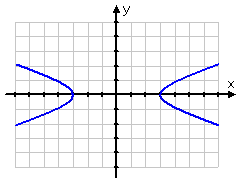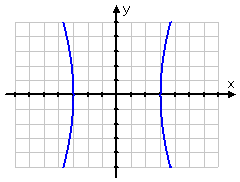The measure of the amount of curvature is the "eccentricity" e, where e = c/a. Since the foci are further from the center of an hyperbola than are the vertices (so c > a for hyperbolas), then e > 1. Bigger values of e correspond to the "straighter" types of hyperbolas, while values closer to 1 correspond to hyperbolas whose graphs curve quickly away from their centers.

Top  |  1 | 2 | 3  |  Return to Index  Next >>

 Cite this article as: Stapel, Elizabeth. "Conics: Hyperbolas: Introduction." Purplemath. Available from     https://www.purplemath.com/modules/hyperbola.htm. Accessed [Date] [Month] 2016

Study Skills Survey

Tutoring from Purplemath
Find a local math tutor